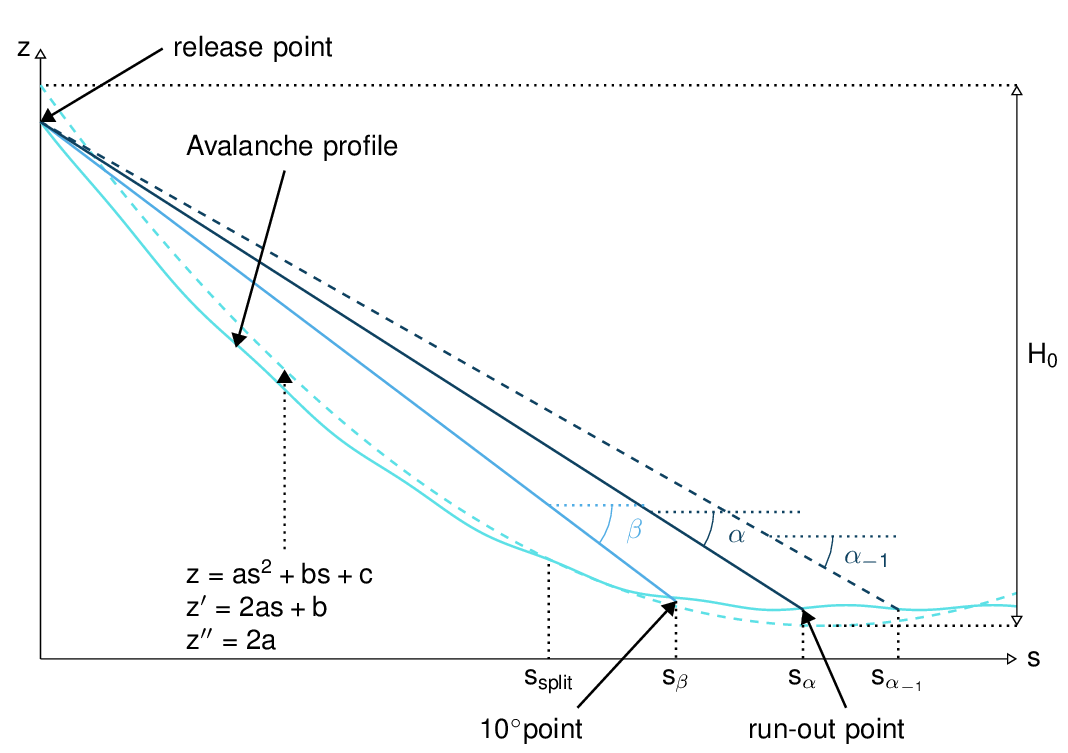# com2AB: Alpha Beta Model¶

com2AB calculates the runout of an avalanche according to the statistical $$\alpha-\beta$$ model. An avalanche is defined by its DEM (digital elevation model), a path and a split point. The runout is calculated according to the $$\alpha-\beta$$ model calibrated for avalanches in the Austrian Alps. It is also possible to adapt the model parameters for other regions.

## Input¶

• raster of the DEM (.asc file)

• set of avalanche paths (shapefile), there can be multiple paths in the shapefile

• Split point (shapefile), there can be multiple split points in the shapefile

## Outputs¶

• profile plot with alpha, beta and runout points

• txt file with angle and coordinates of the different points

## To run¶

• go to AvaFrame/avaframe

• copy com2AB/com2ABCfg.ini to com2AB/local_com2ABCfg.ini and edit (if not, default values are used)

• make sure all the required inputs are available in the avalanche directory

• enter the path to the desired dataset in local_avaframeCfg.ini

• run:

python3 runCom2AB.py


## Theory¶Fig. 1 Topographical variables for the calculation of $$\alpha$$

The snow avalanche runout distance is calculated using a statistical model based on data collected for real avalanches (). An equation of the following type is fitted to the data:

$\alpha_j = k_1 \beta + k_2 z'' + k_3 H_0 + k_4 + j SD$

where $$H_0$$ is the elevation loss of the quadratic fit of the avalanche profile. $$z''$$ is the curvature of this same quadratic fit. $$\beta$$ is the angle of the line between the $$10^\circ$$ point (first point where the avalanche profiles slope is under $$10^\circ$$) and the starting point. The coefficients $$(k_1, k_2, k_3, k_4)$$ and the standard deviation $$SD$$ are calculated during the fitting process. Index $$j=\{-1,-2,0,1\}$$ and $$\alpha_j= \alpha + j SD$$. These coefficients obviously depend on the initial set of chosen data. $$\alpha_0= \alpha$$ is the angle between the stopping and the starting point of the avalanche. $$\alpha_j= \alpha + j SD$$ takes into account the variability of the process. The values of the SD used are based on normal distribution. It is important to note that a bigger runout angle leads to a shorter runout distance. This means that $$\alpha_{-1}= \alpha - SD$$ leads to a longer runout. In other words, the probability of the runout being shorter than $$s_{\alpha_{-1}}$$ corresponding to $$\alpha_{-1}$$ is approximately 83%.

In this module, the coefficients $$(k_1, k_2, k_3, k_4)$$ and the standard deviation $$SD$$ are already known, they are simply used in the $$\alpha$$ equation to calculate the runout on a new profile.

## Procedure¶

Preprocessing :

• The avalanche path (x,y) is first resampled. Default value for resampling is distance=10m (maximal horizontal distance between two points). Note that it does not make much sense to decrease this value to be smaller than the raster grid resolution. We then introduce the curvilinear coordinate s which represents the projected horizontal distance along the path.

• The avalanche path is projected on the DEM to generate the profile using a bi-linear interpolation on the DEM to the point of interest.

• The split point (which is not necessarily given on the avalanche path) is projected on the avalanche path.

From this we obtain the (x,y,z) and (s,z) coordinates of the avalanche profile.

AlphaBeta Model:

• Find the $$10^\circ$$ point from (s,z).

• Calculate $$\beta$$.

• Calculate the $$\alpha_j$$ angles using the adequate standard, small avalanche or custom parameter set.

Postprocessing:

• Plot and save results.

## Configuration parameters¶

distance

resampling distance. The given avalanche path is resampled with a step <= 10m (default).

dsMin

float. Threshold distance [m]. When looking for the beta point make sure at least dsMin meters after the beta point also have an angle bellow 10° (dsMin=30m as default).

smallAva

boolean (False as default) if True apply $$(k_1, k_2, k_3, k_4, SD)$$ set of small avalanches or False, standard avalanches

customParam

boolean (False as default). Enables to choose custom $$(k_1, k_2, k_3, k_4, SD)$$. If True, the values from the configuration file are used

k1

float. Use this value if customParam=True

k2

float. Use this value if customParam=True

k3

float. Use this value if customParam=True

k4

float. Use this value if customParam=True

SD

float. Use this value if customParam=True

PlotPath

Plot Avalanche path on raster; default False

PlotProfile

Plot profile; default False

SaveProfile

Save profile to file; default True

WriteRes

Write result to file: default True